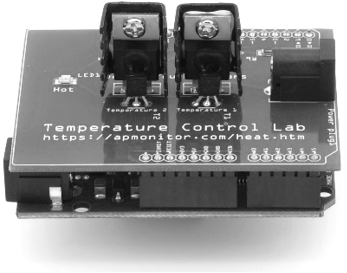## Quiz on Feedforward and Cascade Control1. About how much faster should an inner loop be than an outer loop in cascade control?

A. 2 times as fast
Incorrect. If the inner (secondary) loop is too slow then the primary loop and secondary loop can create an instability. 2x is generally too slow but may work in some cases.
B. 30 seconds faster
Incorrect. The speed of the inner (secondary) loop is relative to the outer loop (primary)
C. At least 3 times as fast
Correct. If the inner (secondary) loop is too slow then the primary loop and secondary loop can create an instability
D. 10 times as fast
Incorrect. It is desirable to have the inner loop faster but 10x is not required

2. For a complicated multilayer cascade control system that is performing poorly, what are some solutions for better performance? Select two possible solutions.

A. Replace all the cascade control with feedforward control
Incorrect. A cascade controller inner loop rejects a disturbance before it can affect the outer loop process variable. It is often preferable to control the disturbance versus compensate with feedforward trim.
Incorrect. More complexity can make the interacting system even worse
C. Decouple interacting controllers with better pairing of actuator and process variable.
Correct. An RGA (Relative Gain Array) can assist in this analysis.
D. Place the primary controller in manual and retune the secondary (inner loop) controller
Correct. This is often effective to reject a disturbance before it affects the primary controller process variable

3. Which of the following is a difference between feedforward and cascade control?

A. Cascade control uses two controllers
Incorrect. The correct answer is D
B. Feedforward uses a disturbance model to determine the feedforward gain
Incorrect. The correct answer is D
C. Cascade has an inner control loop to directly reject disturbances
Incorrect. The correct answer is D
D. All of the above
Correct.

4. Under what circumstances does feedforward control not reject disturbances?

A. Dynamics for the actuator and the process
Incorrect. Transfer functions for the process and actuator are typical. Transfer functions can be combined by multiplying the transfer functions in series.
B. Process time delay is more than the disturbance time delay \theta_p < \theta_d
Incorrect. Feedforward control works well if the process dead time is less than the disturbance dead time
C. Process and disturbance time constants are significantly different \tau_p and \tau_d
Incorrect. This is more challenging, but requires a lead-lag controller G_{ff} instead of a feedforward controller gain K_{ff}
D. Dead time on the process is too great to act in anticipation of the disturbance
Correct. Feedforward control is not effective when \theta_p is greater than \theta_d

5. Given the following two transfer functions for a process and disturbance, find the feedforward control gain K_{ff}.

$$G_p(s) = \frac{7.0e^{-4s}}{5s + 1}$$

$$G_d(s) = \frac{4.0e^{-5s}}{4s + 1}$$

A. 7
Incorrect. This is the process gain

$$K_p = \lim_{s \to 0} G_p(s) = \lim_{s \to 0} \frac{7.0e^{-4s}}{5s + 1} = 7$$

B. -{4}/{7}
Correct.

$$K_p = \lim_{s \to 0} G_p(s) = \lim_{s \to 0} \frac{7.0e^{-4s}}{5s + 1} = 7$$

$$K_d = \lim_{s \to 0} G_d(s) = \lim_{s \to 0} \frac{4.0e^{-5s}}{4s + 1} = 4$$

$$G_{ff} = -\frac{K_d}{K_p} = -\frac{4}{7}$$

C. {5}/{4}
Incorrect. Review the Final Value Theorem (FVT) to find the gain of the process and disturbance
D. {4}/{7}
Incorrect. It is missing the negative sign